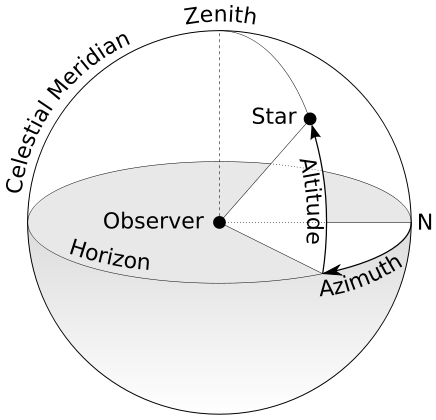# Azimuth Angle

An azimuth (from Arabic al-sumūt, meaning "the directions") is an angular measurement in a spherical coordinate system. The vector from an observer (origin) to a point of interest is projected perpendicularly onto a reference plane; the angle between the projected vector and a reference vector on the reference plane is called the azimuth.An example is the position of a star in the sky. The star is the point of interest, the reference plane is the horizon or the surface of the sea, and the reference vector points north. The azimuth is the angle between the north vector and the perpendicular projection of the star down onto the horizon.